# Visual Notes on Spherical Harmonics

Spherical Harmonics are a core building block of Equivariant Neural Networks. This post breaks them down by analyzing them as 3D extensions of the Fourier Series.
geometry
equivalence
Published

October 10, 2021

Spherical harmonics are powerful mathematical tools, allowing us to represent any function on a sphere as the sum of simpler basis functions (much like a Fourier series!). This post aims to explain spherical harmonics through the lens of Fourier series, by “lifting” them from a circle to a sphere, extensively relying on visual explanations.

Code
import plotly.express as px
from plotly.subplots import make_subplots
import plotly.graph_objects as go

import numpy as np

## Functions on Circles

Suppose we have a periodic function $$f(\theta)$$, with a period $$2\pi$$. As an example, let $$f(\theta) = \sin(\theta) - 0.5\cos(2\theta) + 0.25\sin(3\theta)$$. This is the standard way to view the function: angle $$θ$$ on the x-axis, $$f(θ)$$ on the y-axis.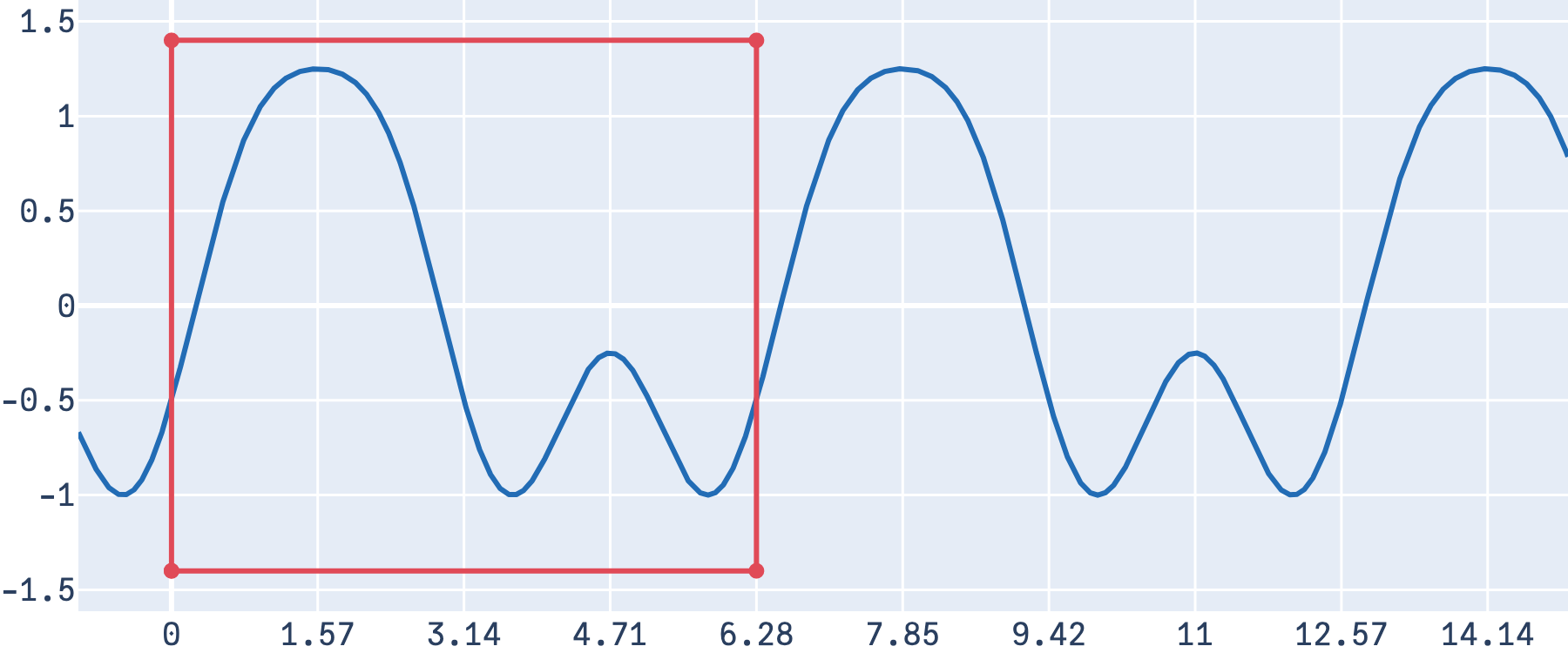The red box denotes one full cycle, which we can see is from 0 to 2π

Since the function is periodic, there is an equivalent way to view this: as a function defined on a circle with radius 1 (and in turn, a circumference of 2π). The period here is represented counter-clockwise (as is convention in trigonometry). Just as in the previous plot, we can see that the function first rises to a large “hill”, before descending into two small “valleys”, returning to the starting point.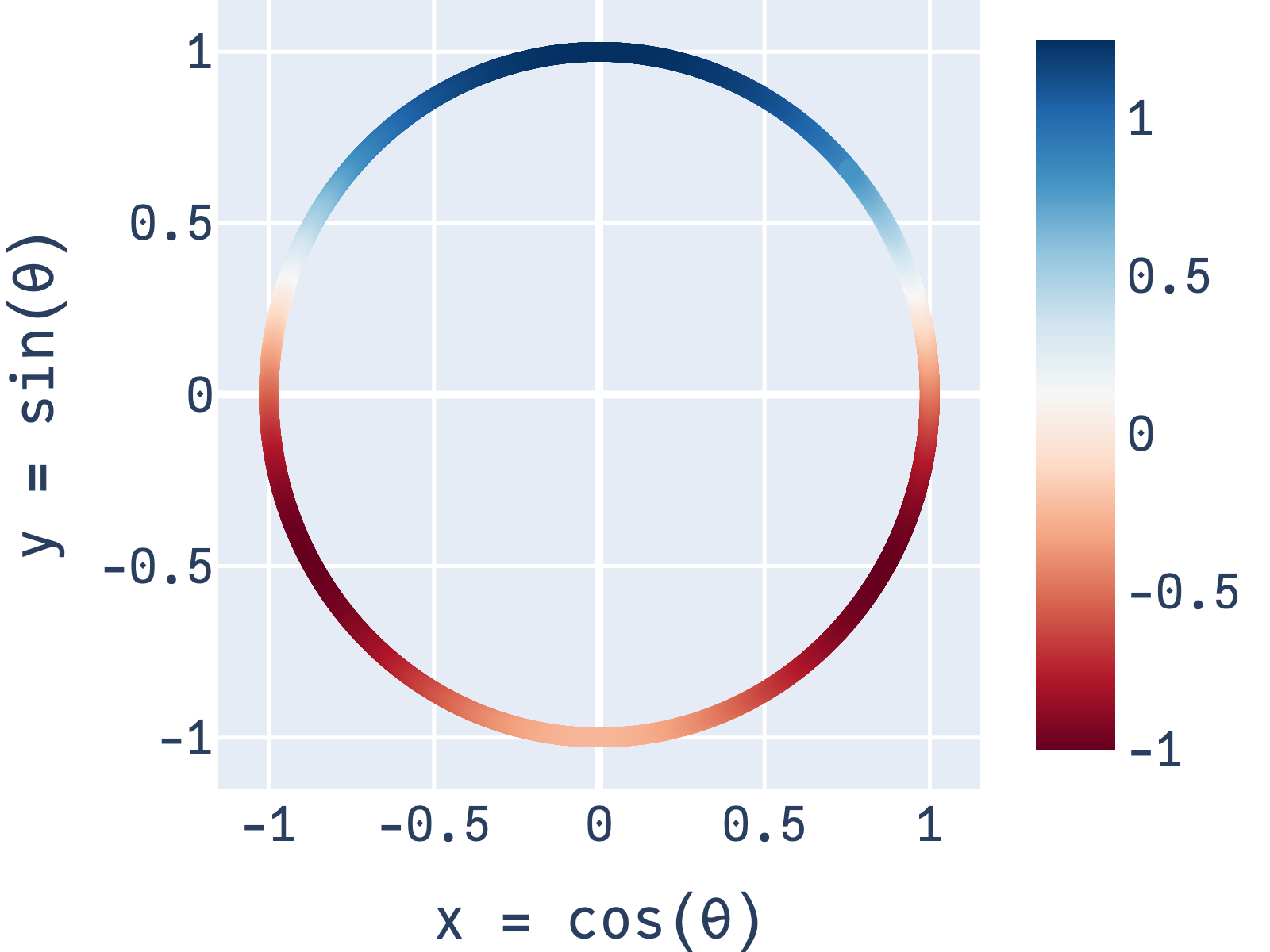Color denotes the value of the function at $$x = cos(θ), y = sin(θ)$$

### Fourier Series

One of the most widely used discoveries in mathematics is that any real-valued, periodic function1 can be represented as the weighted sum of sines and cosines2 of varying frequencies, called a Fourier Series. Specifically, for a function with a period of $$2\pi$$, there exists weights $$a_n$$ (weights for the cosines) and $$b_n$$ (weights for the sines) such that:

$f(\theta) = \frac{a_0}{2} + \sum_{n=1}^\infty a_n \cos(n\theta) + b_n \sin(n\theta)$

The process of finding the exact weights belong to the study of Fourier Analysis. In our case, since the function we’ve chosen is already neatly written as a sum of sines and cosines, $$f(\theta) = \sin(\theta) - 0.5\cos(2\theta) + 0.25\sin(3\theta)$$, the weights can be read off directly: $$b_1 = 1$$, $$a_1 = -0.5$$ and $$b_3 = 0.25$$; all the other weights are zero3. We can also visualize $$f(θ)$$ with the relevant sine and cosine functions (a.k.a the “basis functions”)4.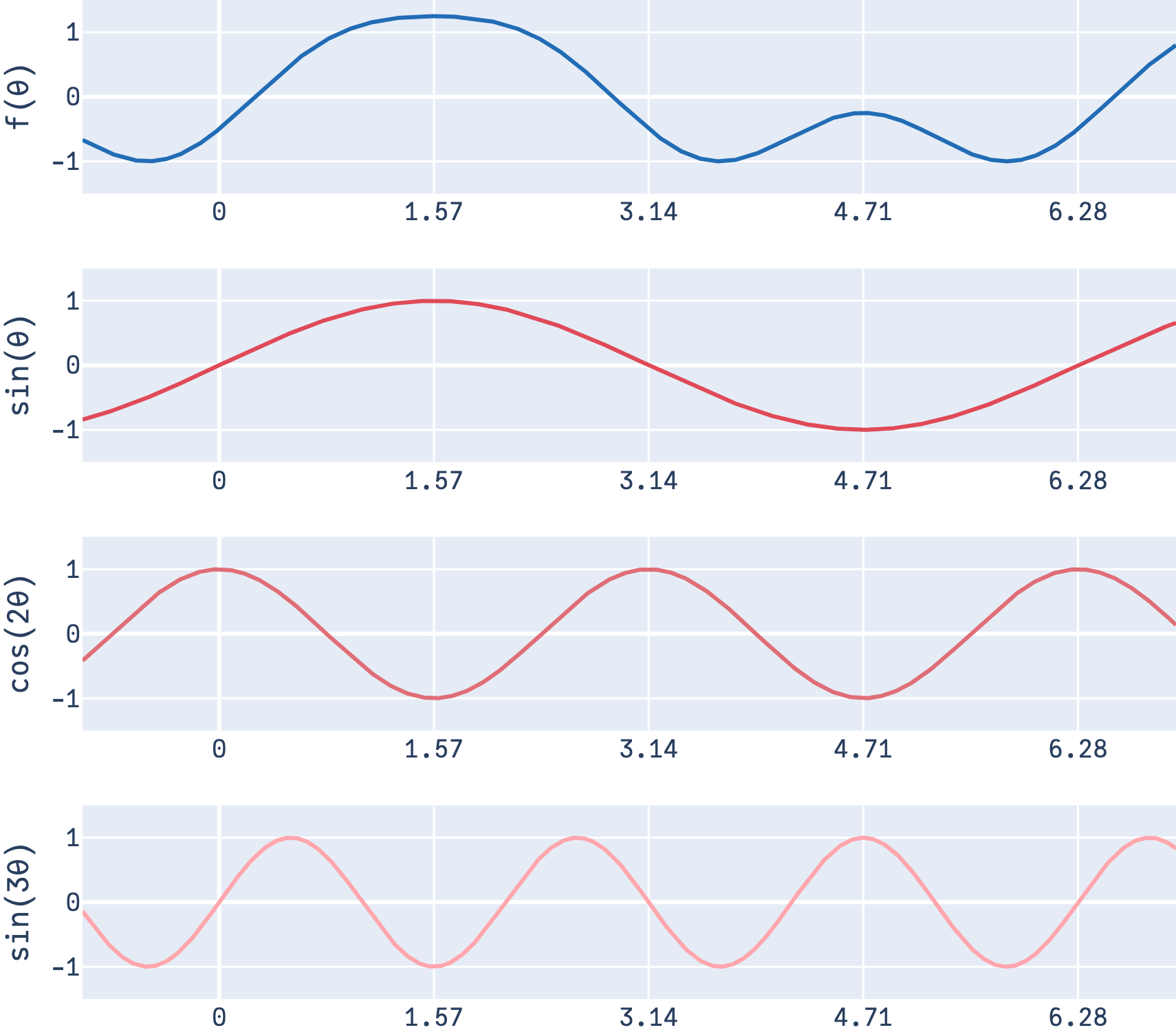The blue function is obtained as a weighted sum of the red functions

Note that for a finite number of terms, this is exact only when the function can be expressed in terms of sines and cosines; otherwise (with function such as say, a square wave) this only exact when the sum uses an infinite number of terms.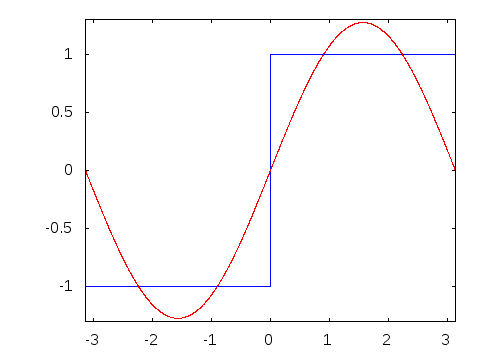The approximation by trigonometric functions becomes increasingly more accurate as more terms are added. (Figure by Thenub314, original here)

Looping back5 to earlier, these basis functions can also be represented on a circle: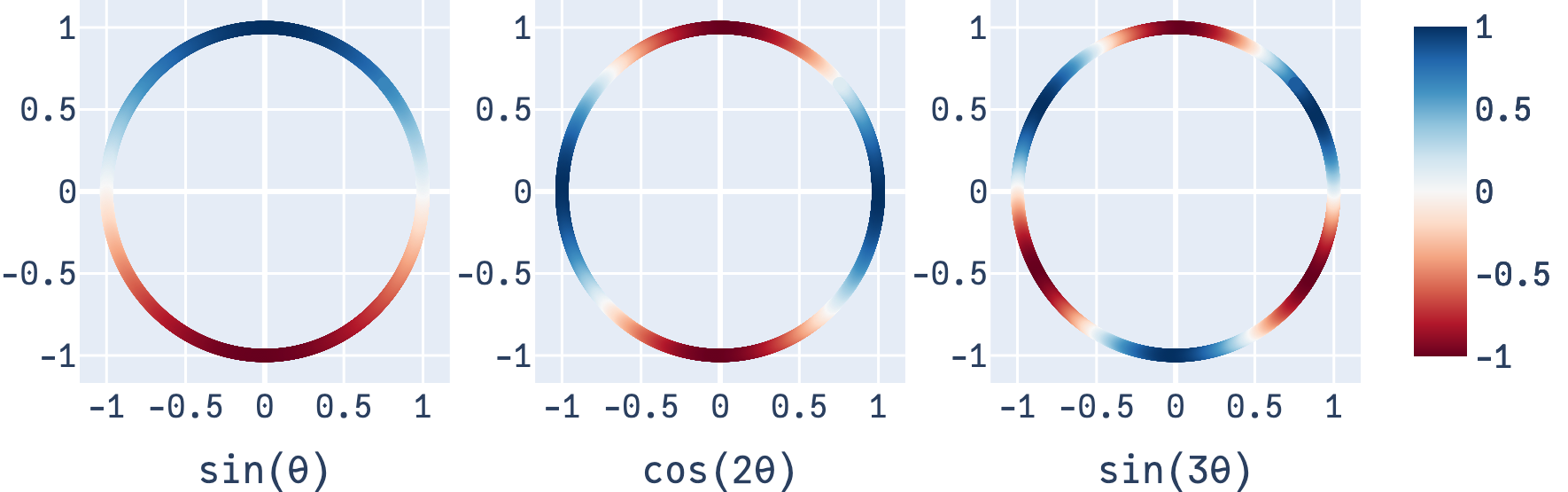As would be expected, $$sin(θ)$$ only finishes one “cycle” on the circle, whereas $$sin(3θ)$$ finishes 3.

The takeaway here is that even though the circle plots look very different from the standard plot (with $$θ$$ on the x-axis), they’re simply two different ways of depicting the exact same function on a circle.

## Spherical Harmonics

If you’ve followed the visuals so far, you already know what a spherical harmonic is: instead of basis functions defined on a circle, they’re basis functions defined on a sphere 6. Just like how $$\sin(\theta)$$ forms a function on a circle, the spherical harmonics are functions on a sphere. Here’s one (it’s interactive!):

Code
fig = make_subplots(rows=1, cols=1, specs=[[{'is_3d': True}]])

s = np.linspace(0, 2 * np.pi, 100)
t = np.linspace(0, np.pi, 100)
tGrid, sGrid = np.meshgrid(s, t)

r = 1
x = r * np.cos(sGrid) * np.sin(tGrid)
y = r * np.sin(sGrid) * np.sin(tGrid)
z = r * np.cos(tGrid)

go.Surface(x=x, y=y, z=z,
surfacecolor=np.sqrt(3/4*np.pi)*y,
colorscale='RdBu',
colorbar=dict(thickness=10)
)
)

fig.update_layout(
font_family="JuliaMono",
showlegend=False,
margin=go.layout.Margin(l=0, r=0, b=0, t=0),
paper_bgcolor='rgba(0,0,0,0)',
)

fig.update_traces(showscale=False)
fig.show()

On the circle, Fourier Analysis allows us to convert any function defined on a circle (i.e. a periodic function) into the weighted sum of sines and cosines of varying frequencies. Now, on the sphere, any function on a sphere can be decomposed into a weighted sum of the spherical harmonic functions $$Y_{lm}$$. That is, there exist $$a_{lm}$$ such that:

\begin{align*} f(x, y, z) &= \sum_{l=0}^\infty \sum_{m=-l}^l a_{lm} Y_{lm}(x,y,z) \\ &\text{where } x^2+y^2+z^2 = 1 \end{align*}

There’s a few things to note here:

• Degree l: Instead of one sum as in a Fourier series, there’s two here. The index of the outer sum, $$l$$ can be thought of as frequency. Just like how $$sin(θ)$$ and $$sin(2θ)$$ refer to one and two cycles on the circle, a harmonic with $$l=1$$ has one cycle, whereas a harmonic with $$l=2$$ has two, as we can see. $$l$$ is the “degree” of the function.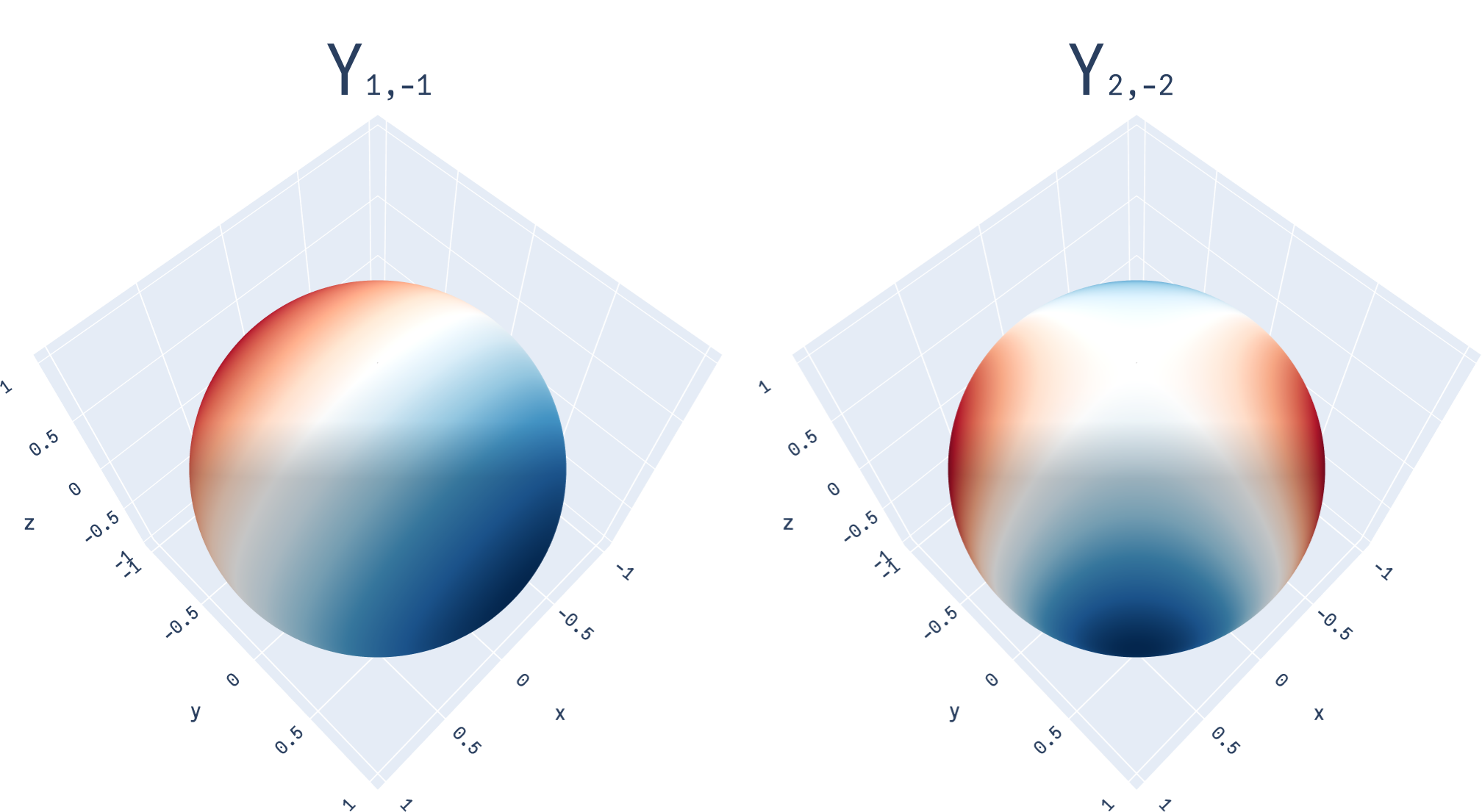One loop around the circle on the x-y plane would result in one “cycle” on left (degree 1), and two on the right (degree 2)
• Order m: The inner sum corresponds to the fact there are $$(2l+1)$$ functions of degree l. (there are 3 functions of degree 1, 5 of degree 2, and so on). This diverges from the Fourier series, where each “frequency” $$n$$ had 2 functions. To distinguish them from each other, each function also has an “order” $$m$$. Here’s all three functions of degree 1: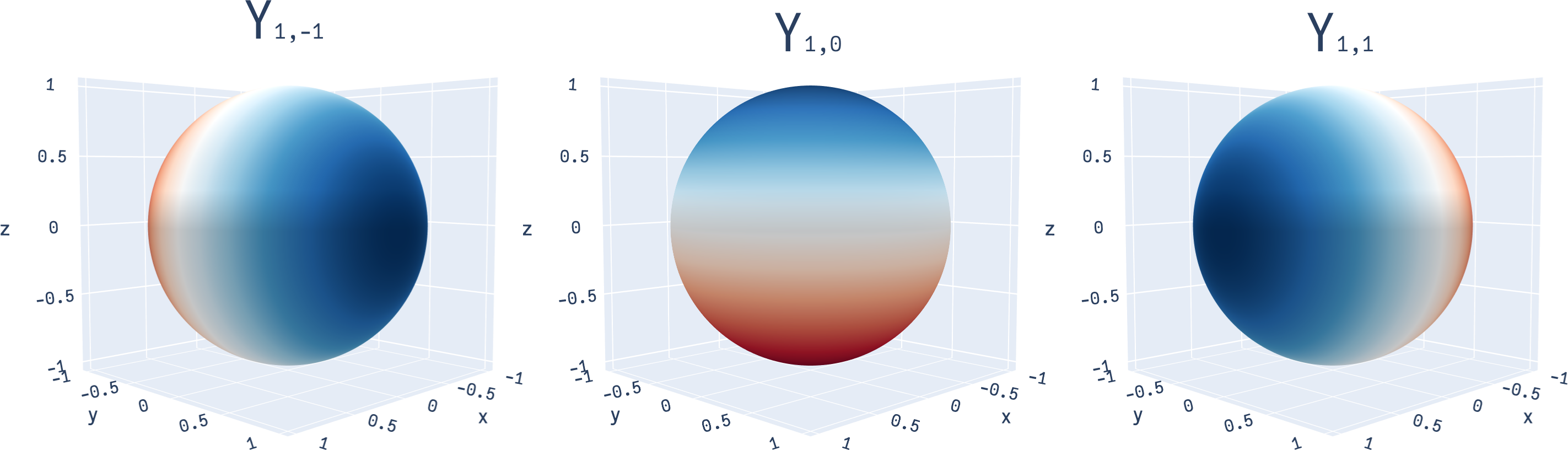The three degree-1 harmonics change across the y, z and x axis respectively. Higher-degree harmonics take on more complex forms.
• Constant term: Just as the Fourier series has the $$\frac{a_0}{2}$$ term (which can be seen as $$\frac{a_0}{2} \cos(0\theta)$$, as $$\cos(0\theta) = 1$$), the spherical harmonics have a constant term in $$a_{00}Y_{00}$$, as the harmonic $$Y_{00}$$ is a function that has the same value everywhere on the circle.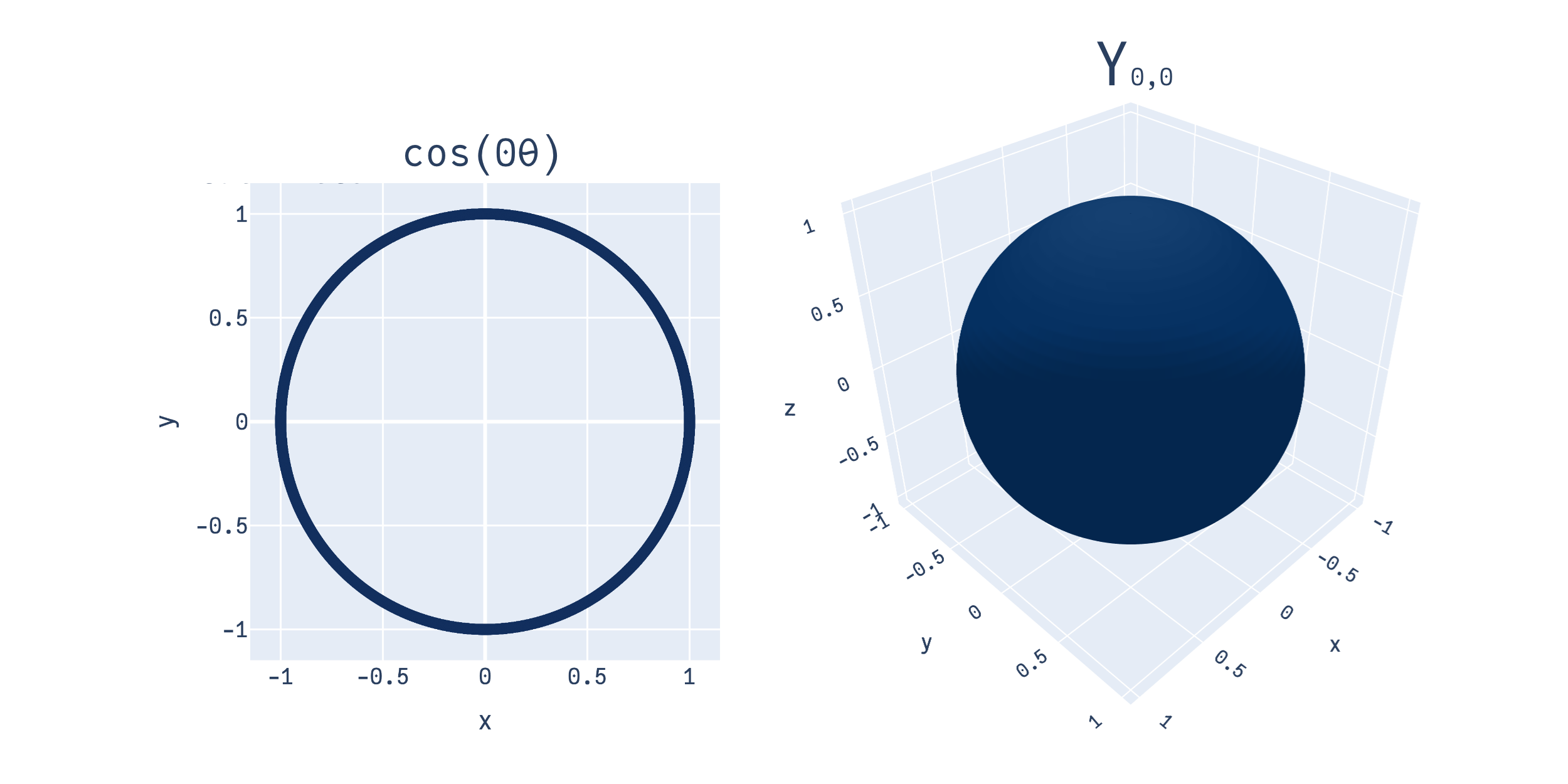Left, we have the “0th” frequency term in the Fourier series. Right, we have the 0th degree spherical harmonic. Both have constant value over their domains.
• Real vs Complex: Both indices are subscripts on $$Y$$, i.e. of the form $$Y_{lm}$$. This convention reflects that we’re looking at the real spherical harmonics here (complex ones have indices of the form $$Y_l^m$$)

• Coordinates: Note that we’re using cartesian coordinates (x, y, z) as inputs to the spherical harmonics, not angles. We could equivalently use harmonics of the form $$Y_{lm}(\theta, \phi)$$ to stay consistent with the Fourier series, but they’re simpler in cartesian form (and as we’ll see in a bit, equivalent).

## Harmonics: Visualization

Often, spherical harmonics are not depicted on the sphere directly, but in a different form. For instance, in the alternate depiction $$Y_{1, -1}$$ looks as such:

Code
fig = make_subplots(rows=1, cols=1,
specs=[[{'is_3d': True}]])

theta = np.linspace(0, np.pi, 100)
phi = np.linspace(0, 2*np.pi, 100)
theta, phi = np.meshgrid(theta, phi)

x = np.sin(theta) * np.cos(phi)
y = np.sin(theta) * np.sin(phi)
z = np.cos(theta)

r0a = np.sqrt(3/4*np.pi)*y
r0 = np.abs(r0a)
go.Surface(
x=r0*x, y=r0*y, z=r0*z,
surfacecolor=r0a,
colorscale='RdBu'
)
)

fig.update_layout(
font_family="JuliaMono",
showlegend=False,
margin=go.layout.Margin(l=0, r=0, b=0, t=0),
paper_bgcolor='rgba(0,0,0,0)',
)

fig.update_layout(
scene = dict(
xaxis = dict(nticks=4, range=[-1.8,1.8],),
yaxis = dict(nticks=4, range=[-1.8,1.8],),
zaxis = dict(nticks=4, range=[-1.8,1.8],)
),
scene1_aspectratio=dict(x=1, y=1, z=1)
)

fig.update_traces(showscale=False)
fig.show()